# A, B, C and D please

Assume that unemployment, u, is related to inflation, π, according to the following Phillips curve: u = u − φ (π−πe), where u is the natural rate of unemployment and πe is the expected rate of inflation. Assume rational expectations and that the central bank’s preferences are given by the loss function L(u,π) =λu+π2, where λ denotes the weight that the central bank assigns unemployment.

a. Suppose that φ = 1. Show what rate of inflation a central bank with λ = .04 will choose under discretion. What will the unemployment rate be?

b. Assume that a less conservative executive board of the central bank is appointed with the weight λ = .08 on unemployment. What is the new inflation rate? How is unemployment affected?

c. What will inflation and unemployment be if the executive board of the central bank does not care at all about unemployment, i.e. if λ = 0?

d. What can we learn from the calculations above?

Solution:-

Given that

As given in the question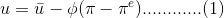where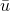= Natural rate of unemployment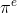= Expected rate of inflation.

u = Actual unemployement Rate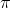= Actual inflation rate

Equation (1) can be written as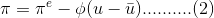when \pi =1 , then Actual inflation rate depends directly on past year inflation rate and in that case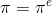,

Now '\lambda' which is the weight assign to unemployment depends on actual inflation and '1-\lambda' depends on expected inflation.

So, eqn (2)

becomes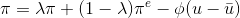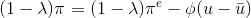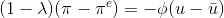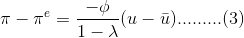a)

When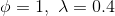As we know when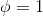, thenso from eqn (1)

we get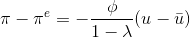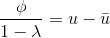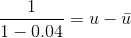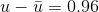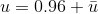unemployement rate will be 0.96 more than natural rate of unemployement

b)

When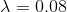then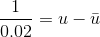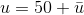unemployment rate will be 50 more than natural rate of unemployment.

Inflation will still depend on part year inflation asc)

When 0

Then from eqn (3)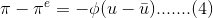From eqn (4) it follow that change in inflation rate depends on deviation of unemployment rate from natural rate and its magnitude is determined by the values of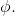d)

The above calculation show that, in case of high inflation, \phi is positive and the relation between unemployment rate and inflation rate is likely to change, as a result of which the manner in which expectation are formed get changed.

Thanks for supporting***

Similar Homework Help Questions

Assume that unemployment, u, is related to inflation, π, according to the following Phillips curve: u = u − φ (π−πe), where u is the natural rate of unemployment and πe is the expected rate of inflation. Assume rational expectations and that the central bank’s preferences are given by the loss function L(u,π) =λu+π2, where λ denotes the weight that the central bank assigns unemployment.A. Suppose that φ = 1. Show what rate of inflation a central bank with λ...

• ### i only need question 5, thanks 4. Suppose that the Phillips curve is given by TT,...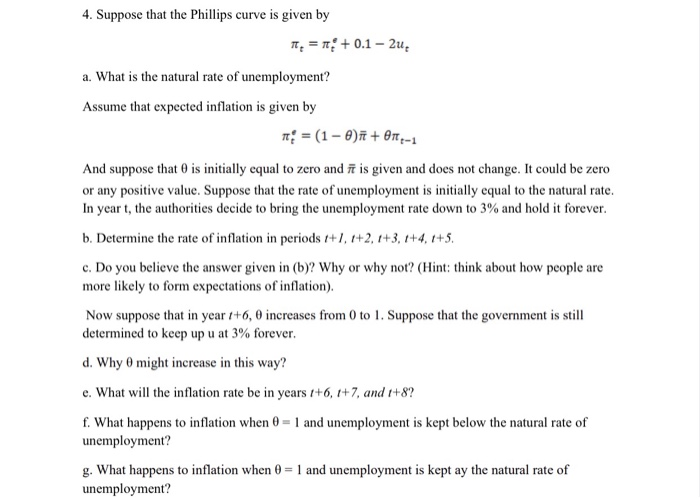i only need question 5, thanks 4. Suppose that the Phillips curve is given by TT, = 1 + 0.1 - 2u a. What is the natural rate of unemployment? Assume that expected inflation is given by m = (1 - 0) +010-1 And suppose that is initially equal to zero and it is given and does not change. It could be zero or any positive value. Suppose that the rate of unemployment is initially equal to the natural rate....

• ### Suppose that the Phillips curve is given by - = 0.1 - 2u where = -1 Suppose that inflation in year t - 1 is ze...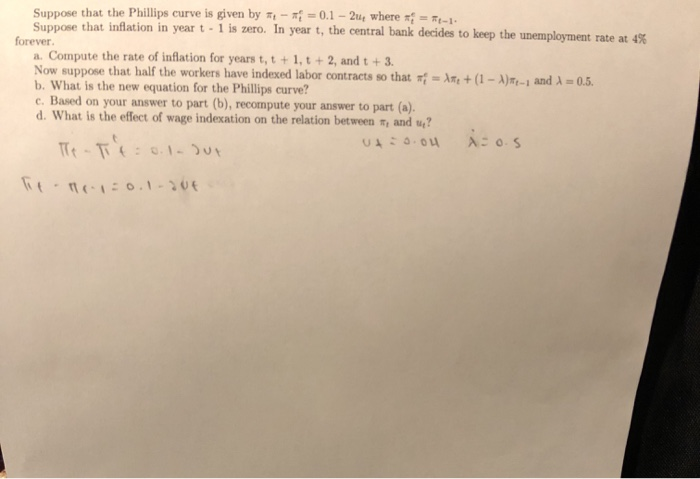Suppose that the Phillips curve is given by - = 0.1 - 2u where = -1 Suppose that inflation in year t - 1 is zero. In year t, the central bank decides to keep the unemployment rate at 45 forever a. Compute the rate of inflation for years t, t + 1, t + 2, and t + 3. Now suppose that half the workers have indexed labor contracts so that = + (1 - A)-1 and 1 =...

• ### True, False or Uncertain (24 points). State whether the claims in these statements are true, false...

True, False or Uncertain (24 points). State whether the claims in these statements are true, false or ambiguous and explain why. You must provide an explanation to receive any credit. Drawing graphs and arrows only will yield minimum credit. a. “In the Mundell-Fleming (IS*-LM*) Model with fixed exchange rates, a contractionary monetary policy will have no effect on output in the long-run.” (8 points) b. ““Suppose that the equation for the Phillips curve is π = πe – 3(u -...

• ### 3. Discuss the relationship between the natural rate of unemployment, Un, and the Phillips curve, 1lt...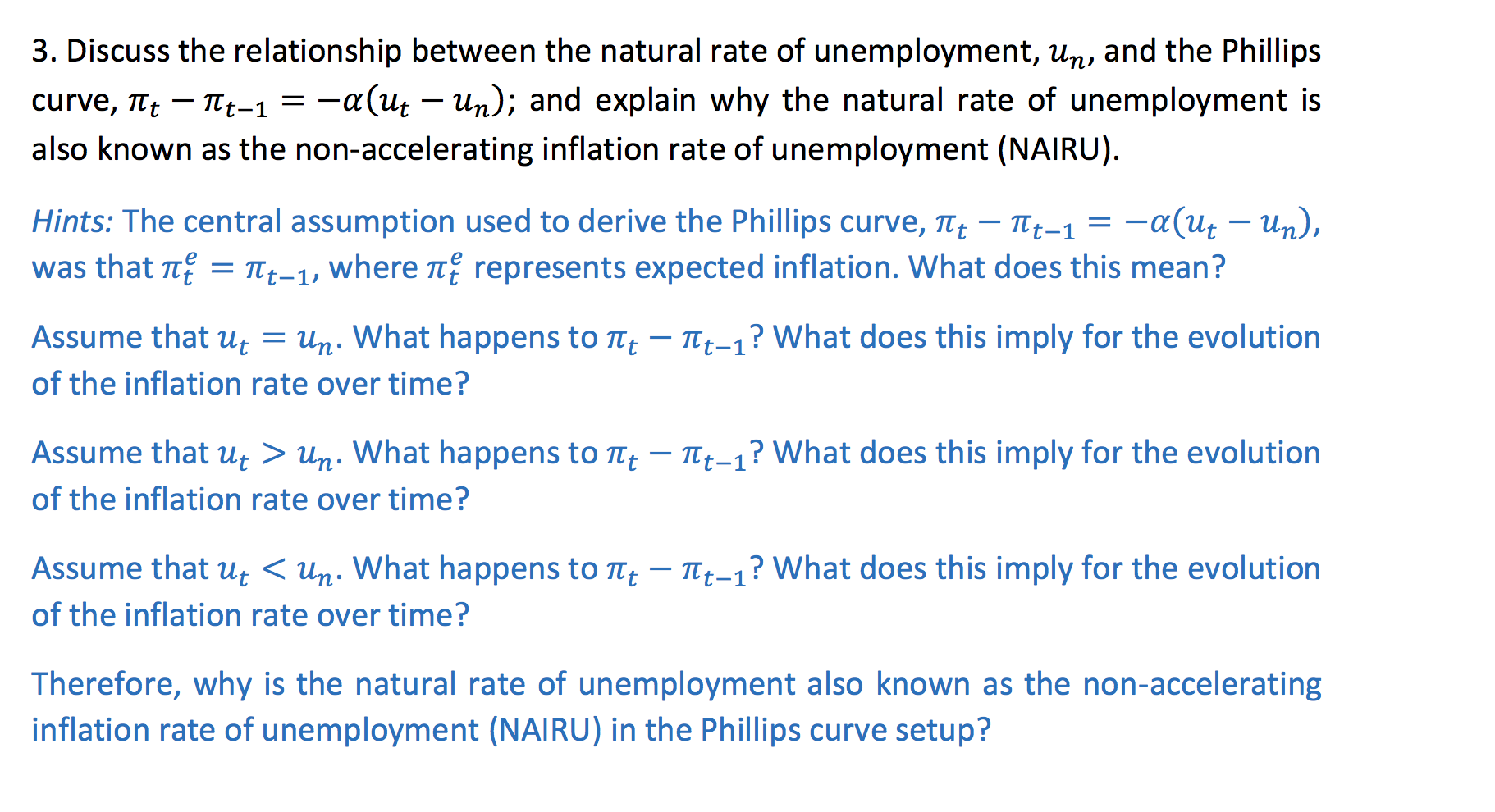3. Discuss the relationship between the natural rate of unemployment, Un, and the Phillips curve, 1lt – itt-1 = -a(ut – Un); and explain why the natural rate of unemployment is also known as the non-accelerating inflation rate of unemployment (NAIRU). Hints: The central assumption used to derive the Phillips curve, Tet – 1lt-1 = -a(Ut – Un), was that tę = Tt-1, where tę represents expected inflation. What does this mean? Assume that Ut = Un. What happens to...

• ### Suppose the central bank, instead of following the rule r = r(Y,π), has a target level...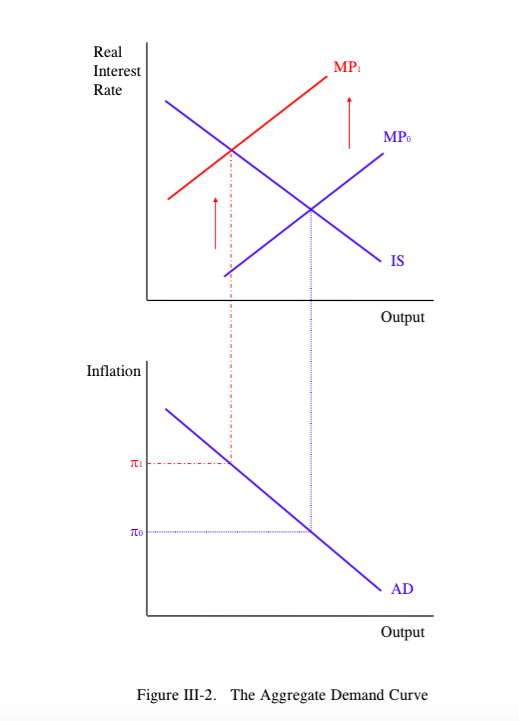Suppose the central bank, instead of following the rule r = r(Y,π), has a target level of inflation. Specifically, it sets r according to r = rLR + b[π − π*]. Here rLR is the real interest rate when the economy is in long-run equilibrium; that is, it is the real interest rate that causes the loan market to be in equilibrium when Y = �Y. In addition, π* is the central bank’s target level of inflation, and b is...

• ### 4. (2.5 PTS) Assume the following Phillips curve: where π is the inflation rate, π et...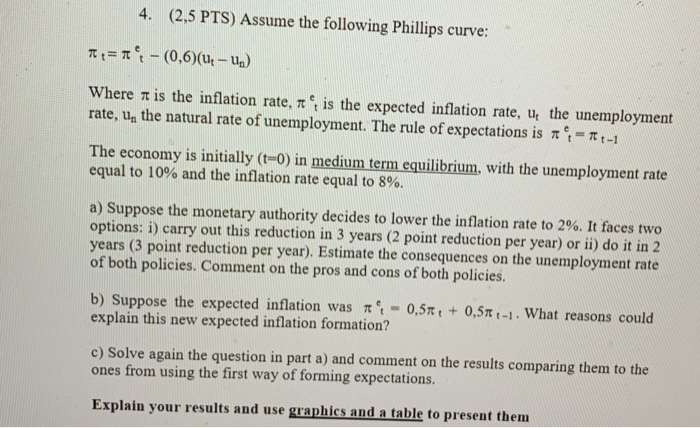4. (2.5 PTS) Assume the following Phillips curve: where π is the inflation rate, π et is the expected inflation rate, ut the unemployment rate, un the natural rate of unemployment. The rule of expectations is π e.-π t-1 The economy is initially (t-0) in medium term equilibrium, with the unemployment rate equal to 10% and the inflation rate equal to 8%. a) Suppose the monetary authority decides to lower the inflation rate to 2%. It faces two options: i)...

• ### Question 1 (42 p) Consider a closed economy where goods market and finalcial markets can be descr...Question 1 (42 p) Consider a closed economy where goods market and finalcial markets can be described by the following equations for period t С,-100 + 0.5yo- 2000.25Y- 200r G- 100: T-200 Suppose inflation escpectations in this economy is based on past period's inflation rate, ie. Let Yo- FIN)-No the labor force is given as constant at LF- 1000. (4p) Write down the IS equation for this economy (4p) Assume a horizontal LM function where the Central Bank announces the...

• ### Question 1 Assume that inflation and social welfare are given by r = ne-au- u*) and...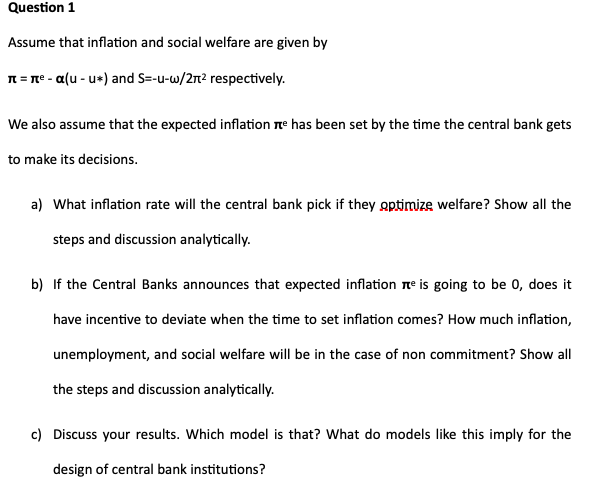Question 1 Assume that inflation and social welfare are given by r = ne-au- u*) and S=-u-w/212 respectively. We also assume that the expected inflation ne has been set by the time the central bank gets to make its decisions. a) What inflation rate will the central bank pick if they optimize welfare? Show all the steps and discussion analytically. b) If the Central Banks announces that expected inflation ne is going to be 0, does it have incentive to...

• ### 2. Phillips Curve. An economy has the following functions for its short run aggregate supply (SRAS),...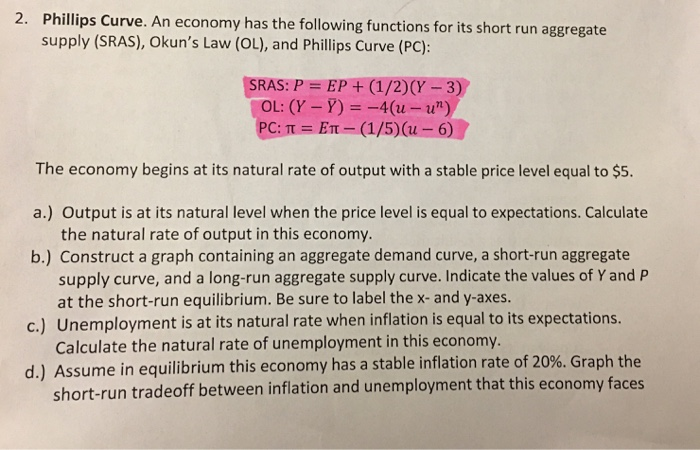2. Phillips Curve. An economy has the following functions for its short run aggregate supply (SRAS), Okun's Law (OL), and Phillips Curve (PC): SRAS: P = EP + (1/2)(y - 3) OL: (Y-Y) = -4(u-u") PC:T = ET - (1/5)( - 6) The economy begins at its natural rate of output with a stable price level equal to \$5. a.) Output is at its natural level when the price level is equal to expectations. Calculate the natural rate of output...

Free Homework App